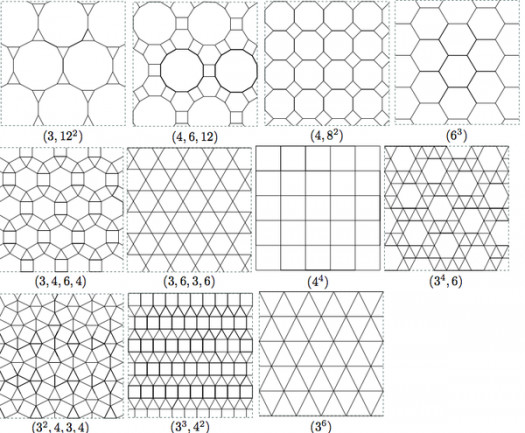# Do You Know Much About Negative Exponents?

Approved & Edited by ProProfs Editorial Team
At ProProfs Quizzes, our dedicated in-house team of experts takes pride in their work. With a sharp eye for detail, they meticulously review each quiz. This ensures that every quiz, taken by over 100 million users, meets our standards of accuracy, clarity, and engagement.
| Written by Livyn
L
Livyn
Community Contributor
Total Contribution - 348 | Total attempts - 140,973
Questions: 10 | Attempts: 166SettingsA negative exponent just means that the base is on the wrong side of the fraction line, so you need to flip the base to the other side.

• 1.

### Negative exponents is topic in ...

• A.

Mathematics

• B.

Music

• C.

Sociology

• D.

Biology

A. Mathematics
Explanation
Negative exponents is a topic in Mathematics. In mathematics, an exponent represents the number of times a base is multiplied by itself. A negative exponent indicates the reciprocal of the base raised to the positive exponent. Understanding negative exponents is important in various mathematical concepts and calculations, such as simplifying expressions, solving equations, and working with scientific notation.

Rate this question:

• 2.

### Mathematics is usually studied in ...

• A.

Mosque

• B.

School

• C.

Home

• D.

Church

B. School
Explanation
Mathematics is usually studied in school because it is a fundamental subject taught in educational institutions. Schools provide a structured curriculum and qualified teachers who can effectively teach mathematical concepts and theories. Moreover, schools also offer resources such as textbooks, study materials, and mathematical tools that facilitate the learning process. Additionally, schools provide an environment that encourages interaction and collaboration among students, which is beneficial for learning mathematics through group discussions and problem-solving activities. Therefore, studying mathematics in school is the most logical and practical option.

Rate this question:

• 3.

### Who among them is not a mathematician?

• A.

Pythagoras

• B.

Isaac Newton

• C.

Albert Einstein

• D.

Will Smith

D. Will Smith
Explanation
Will Smith is not a mathematician because he is a well-known actor and musician, not a mathematician. Pythagoras, Isaac Newton, and Albert Einstein are all famous mathematicians who have made significant contributions to the field of mathematics.

Rate this question:

• 4.

### Exponents are also called ....

• A.

Powers

• B.

Ratio

• C.

Decimals

• D.

Equations

A. Powers
Explanation
Exponents are also called powers because they represent the number of times a base number is multiplied by itself. In an exponent, the base number is raised to the power or exponent, resulting in a new value. This concept is commonly used in mathematics and helps simplify calculations and express large or small numbers more conveniently. Therefore, the term "powers" accurately describes the relationship between the base number and the exponent.

Rate this question:

• 5.

### A negative exponent means how many timesto .... by the number.

• A.

Multiply

• B.

Divide

• C.

• D.

Subtract

B. Divide
Explanation
A negative exponent means how many times to divide by the number. When a number is raised to a negative exponent, it is equivalent to taking the reciprocal of the number and raising it to the positive exponent. This means that the number is divided by itself raised to the positive exponent. Therefore, the correct answer is divide.

Rate this question:

• 6.

### The exponent of a number says how many timesto use the number in a ....

• A.

• B.

Subtraction

• C.

Division

• D.

Multiplication

D. Multiplication
Explanation
The exponent of a number indicates how many times the number should be multiplied by itself. For example, if a number is raised to the power of 2, it means that the number should be multiplied by itself once. If it is raised to the power of 3, it should be multiplied by itself twice. Therefore, the correct answer is multiplication, as the exponent determines the number of times multiplication should be performed.

Rate this question:

• 7.

### Negative Exponents is under ....

• A.

Proportions

• B.

Exponents

• C.

Decimals

• D.

Equations

B. Exponents
Explanation
Negative exponents are a concept in mathematics that falls under the topic of exponents. Exponents involve raising a number to a certain power, and negative exponents indicate the reciprocal of that number. For example, x^(-2) is the same as 1/x^2. Therefore, negative exponents are a specific aspect of the broader concept of exponents.

Rate this question:

• 8.

### Exponents is a topic under ....

• A.

Algebra

• B.

Geometry

• C.

Decimals

• D.

Cube Roots

A. Algebra
Explanation
Exponents is a topic under Algebra because it involves the use of mathematical operations to represent repeated multiplication. In algebra, exponents are used to simplify expressions, solve equations, and represent quantities raised to a power. Understanding exponents is essential in algebraic manipulations and helps in solving various algebraic problems.

Rate this question:

• 9.

### Algebra is a topic under which subject?

• A.

Music

• B.

Biology

• C.

Creative Arts

• D.

Mathematics

D. Mathematics
Explanation
Algebra is a branch of mathematics that deals with symbols and the rules for manipulating those symbols. It is used to solve mathematical equations and represent relationships between variables. Therefore, the correct answer is Mathematics.

Rate this question:

• 10.

### Mathematics is studied by ....

• A.

Humans

• B.

Toys

• C.

Aliens

• D.

AnimalsBack to top